﻿ The Modified Resonant Method for Measuring the Velocity Factor of the Electromagnetic Wave in the Microstrip Transmission Line

### The Modified Resonant Method for Measuring the Velocity Factor of the Electromagnetic Wave in the Mi...

Alexey Kopylov, Yuri Salomatov, Alexander SenchenkoOPEN ACCESSPEER-REVIEWED

## The Modified Resonant Method for Measuring the Velocity Factor of the Electromagnetic Wave in the Microstrip Transmission Line

Alexey Kopylov1,, Yuri Salomatov1, Alexander Senchenko1

1Radio Engineering Department of the Engineering Physics and Radio Electronics Institute of the Siberian Federal University, Krasnoyarsk, Russia

### Abstract

To measure the real value of velocity factor of the electromagnetic waves in the microwave microstrip transmission line was developed a modified resonant method and implements its measuring system. This system is using measuring the resonant frequency of a closed on the end one wave microstrip resonator. The method is designed to determine the velocity factor of the electromagnetic wave in the microstrip transmission line on substrates “policor” (95% Al2O3) for a small batch of microwave hybrid integrated circuits.

### At a glance: Figures

1
Prev Next

• Kopylov, Alexey, Yuri Salomatov, and Alexander Senchenko. "The Modified Resonant Method for Measuring the Velocity Factor of the Electromagnetic Wave in the Microstrip Transmission Line." Journal of Instrumentation Technology 2.1 (2014): 5-8.
• Kopylov, A. , Salomatov, Y. , & Senchenko, A. (2014). The Modified Resonant Method for Measuring the Velocity Factor of the Electromagnetic Wave in the Microstrip Transmission Line. Journal of Instrumentation Technology, 2(1), 5-8.
• Kopylov, Alexey, Yuri Salomatov, and Alexander Senchenko. "The Modified Resonant Method for Measuring the Velocity Factor of the Electromagnetic Wave in the Microstrip Transmission Line." Journal of Instrumentation Technology 2, no. 1 (2014): 5-8.

 Import into BibTeX Import into EndNote Import into RefMan Import into RefWorks

### 1. Introduction

Although the current trend in the development of the microwave (MW) technology is a massive shift from hybrid integrated circuits (HIS) to microwave monolithic integrated circuit (MIC), many problems arising in the construction of microwave devices of various functional purposes, can still be successfully solved using conventional HIS technology microwave. It is typical for microwave technology decimeter and centimeter wavelengths, where the dimensions of the elements of transmission lines in free space is very large and this requires use as a constructive basis HIS microwave substrates with sufficiently large value of the relative permittivity. These devices can include filters , the matching circuit , microwave transfer circuits of energy and signals , as well as a microwave device other than radio, assignments . At design and realization of such devices based on microwave HIS the recurrent problem is the more or less accurate determination of basic characteristics used in this dielectric substrates – their permittivity. Knowledge of this value is the key to building, for example, frequency selective microwave devices, where even small mistakes in the definition (in the third decimal place) lead to a substantial care device parameters from those projected. It is not possible to achieve the basic design parameters of these devices – the bandwidths of passband or stopband frequencies and required parameters of amplitude-frequency (AFC), phase-frequency (PFC) characteristics and the characteristics of group delay time (GDT). Thereby, the determination of the permittivity of dielectric substrates for microwave HIS is relevant in our time the widespread introduction of monolithic microwave circuits. Task carried out in the present work was to study the measurement of the permittivityof the substrate to create “policor” (95% Al2O3) frequency selective devices used in HIS of decimeter wavelength range.

### 2. Resonant Method

2.1. Measurement System

Modern systems for measuring characteristics of the dielectric substrates in the microwave range, as a rule, contain a vector network analyzer include a microwave circuit, the processing results of two-port scattering parameter measurements at microwave frequencies (so-called "technology network analysis"). With this approach becomes unimportant, which parameter of dielectric will be calculated (calculated, to recover from the values of the scattering matrix) vector analyzer – the complex dielectric constant or magnetic, or their components. The accuracy of measurement in this case is a few percent over a very wide frequency bands. However, these systems have a significant disadvantage – they are very expensive (price level device – hundreds of thousands of dollars), making them inaccessible in practice. Due to the high cost of measuring systems, which include vector network analyzers firms – manufacturers instrumentation produce cheaper meters dielectric parameters, the principle of which is described as "impedance analysis". The one of the typical examples of such meters can serve as instrument E4991A-002 of Agilent Technologies company . The essence of the impedance analysis is to measure the reactance (conduction) parallel plate capacitor formed by the cylindrical clamping device, with further calculation of the resulting capacitor capacitance (so this method is also called capacitive). With the appropriate processing of the measurements can be active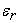and reactive componentsof the complex dielectric constant of the dielectric. However, the magnitude of measurement errors impedance (capacitance) using vary within wide limits and may be from 0,1 % to 90 % depending on the measured permittivity values of dielectric and the ratio of the thickness to the size of the gap .

Due to the fact that former of microwave devices should not know the value of the permittivityof the substrate, but he must know the value of the electromagnetic wave velocity factor in a transmission line, realized on the substrate, we cannot directly measure the valueand use magnitude for practical developments "velocity factor" of electromagnetic wave in a transmission line. The magnitude called the "velocity factor" is the square root of the effective dielectric constant, which indicates how many times the wavelength of the electromagnetic wave in transmission line is reduced in relation to the wavelength in free space. To determine the velocity factor, in our opinion, the most effective method is "resonant method", which is based on the change in resonant frequency when changing the electrical properties of materials.

The studies were conducted in the following order. In the beginning we determined the dielectric constant εAg of the substrate material "polycor" by using the impedance method (capacitive) with use instrument E4991A-002 manufacturer Agilent Technologies  on the frequency 1 GHz. Results showed that they essentially depend on the clamping force of the sample in the measurement system and differ considerably from the passport data. For this reason we decided to measure the velocity factor of an electromagnetic wavein a microstrip transmission line using resonator method  instead of measuring the permittivity of the substrates by using the impedance (capacitive) method .

As a basis we took the known resonance measurement method, which is described in . We have developed a measurement system containing a metal base, on which is attached measured dielectric substrate. On the dielectric substrate superimposed the measuring microstrip resonator, which is pressed against the substrate with a calibrated weight. Resonator configured as a ring to eliminate the influence on the measurement results of the end capacity. The measuring resonator is formed on Foiled Dacron LFR 50-018 (thickness 0.1 mm and the relative permittivity of about 2). Importantly that the permittivity of Dacron significantly below the expected value of the measured permittivity dielectric substrates (for "polycor" this value is about 9). The resonant frequency of the measurement resonator was chosen 1 GHz (this is the frequency limit of the capacitive method implemented for the device E4991A-002 of Agilent Technologies company). The measurements of the resonant frequency of the measuring microstrip resonator was performed on the scalar network analyzer P2M-04 manufactured by CJSC "SPC" Mikran "(Tomsk town of Russia).

Figure 1 shows a photograph of our measuring system. It marked: 1 – the laptop to display the results of measurements; 2 – the scalar network analyzer R2M-04; 3 – the measuring model; 4 – the SWR sensor ДГ1-04-01R; 5 – the head detector Д32-04; 6 – calibrated weight.

Purpose of equipment elements 1-5 in Figure 1 of our measuring system is well known. The purpose of cargo 6 is to reduce the influence of air gaps in a layered design metal base-measured dielectric substrate-measuring resonator. A special place occupies the process of selection quantities of cargo weight 6. Weight of cargo 6 is selected so that the resonance frequency was as low as possible. In this case, the resonator will be most tightly and uniformly adhere to the surface of the substrate and the air layer between them will be minimal. Thus, we expect that at this value of load weight 6 on the resonator obtained the resonant frequency will be closest to the truth.

Purpose of equipment elements 1-5 in Figure 1 of our measuring system is well known. The purpose of cargo 6 is to reduce the influence of air gaps in a layered design metal base-measured dielectric substrate-measuring resonator. A special place occupies the process of selection quantities of cargo weight 6. Weight of cargo 6 is selected so that the resonance frequency was as low as possible. In this case, the resonator will be most tightly and uniformly adhere to the surface of the substrate and the air layer between them will be minimal. Thus, we expect that at this value of load weight 6 on the resonator obtained the resonant frequency will be closest to the truth.

2.2. The Measurements Results

Influence of cargo weight 6 (Figure 1) on resonant frequency of the measuring resonator are demonstrate Figure 2 and Figure 3. On these figures shows the measured dependences of the modulus of transmission coefficient (lower curves) and modulus of reflection coefficient (upper curves) from frequency for measuring resonator with different values of the load weight (pressing force).

Figure 2 shows the frequency dependences of the measuring resonator when installing the first load weight of 1 kg, Figure 2 – when installing the second load weight 2 kg. A further increase in cargo weight, according to our observations, did not cause a significant change in the resonance frequency of the measuring resonator.

Figure 2. Experimental frequency dependences of the measuring resonator when installing the first load weight of 1 kg: upper curve – modulus of the reflection coefficient, the lower curve – modulus of the transfer coefficient
Figure 3. Experimental frequency dependences of the measuring resonator when installing the first load weight of 2 kg: upper curve – modulus of the reflection coefficient, the lower curve – modulus of the transfer coefficient

The results show significant differences in the values of the velocity factor of an electromagnetic wave in a transmission line for the three values of shortening this quantity: for the velocity factor of the length of the electromagnetic wave in the line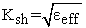obtained in the present study the resonance method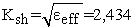, for the expected value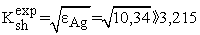, and the velocity factor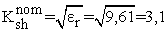is obtained from the nameplate data on the substrate, which can be considered maximum possible value for the substrate. Such a large difference in the values of the coefficients of shortening is likely to involve the use of significantly different between methods of measuring the relative permittivity of the material under study. Furthermore, for different applications must be used different measured parameters of materials. For example in the science of materials must be used measuring of the relative permittivity(these include passport data value and the measurement results of the same magnitudeinstrument Agilent E4991A-002). The constructive-technological and topological aspects of materials used in these devices in this case are not considered.

It is the most best solution that the velocity factor of an electromagnetic wave length in the line will be measured because exactly it magnitude is used in microwave technique for practical realization of the devices based on these or other dielectric materials.

The difference in the velocity factor and relative dielectric constant values for the case of using expected (passport) valueand the case of measure the relative dielectric constant valueby use instrument Agilent E4991A-002 and the case of measure by use our resonant method valuealso conventional by the structure of our resonator. This is due to its rectangular topology and proximity of conducting microstrips polikor the edge of the substrate or the practical realization of the devices based on the used dielectric material is the best measured velocity factor magnitude of an electromagnetic wave in the line because this value is heavily dependent on the design parameters of the microwave device.

### 3. Conсlusion

We believe that the practical use should primarily take into account the magnitude of the velocity factor, obtained by the resonant method measuring.

### References

  Jin, C., Chen, Z. “Compact Triple-Mode Filter Based on Quarter-Mode Substrate Integrated Waveguide”, IEEE Transactions on Microwave Theory and Techniques, 62 (1). 37-45. Jun. 2014.In article CrossRef  Chretiennot, T., Dubuc, D., Grenier, K. “A Microwave and Microfluidic Planar Resonator for Efficient and Accurate Complex Permittivity Characterization of Aqueous Solutions”, IEEE Transactions on Microwave Theory and Techniques, 61 (2). 972-978. Feb. 2013.In article CrossRef  Farzami, F., Norooziarab, M. “Experimental Realization of Tunable Transmission Lines Based on Single-Layer SIWs Loaded by Embedded SRRs”, IEEE Transactions on Microwave Theory and Techniques, 61 (8). 2848-2857. Aug. 2013.In article CrossRef  Kirshin, E., Oreshkin, B., Zhu, G.K., Popovic, M., Coates, M. “Microwave Radar and Microwave-Induced Thermoacoustics: Dual-Modality Approach for Breast Cancer Detection”, IEEE Transactions on Biomedical Ingineering, 61 (2). 354-360. Feb. 2013.In article CrossRef  Agilent. Solutions for Measuring Permittivity and Permeability with LCR Meters and Impedance Analyzers. Application Note 1369-1.In article  Bernard, P. A., Gautray, J. M. “Measurement of Dielectric Constant Using a Microstrip Ring Resonator”, IEEE Transactions on Microwave Theory and Techniques, 32 (3). 974. March 1991.In article